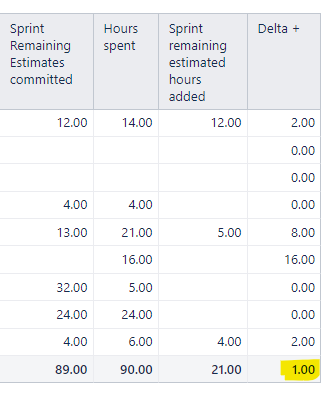# Total is wrongly calculated

Hi,
I’ve prepared measure Delta+ to calculate time of overloaded issues (to find how much):

``````CASE WHEN
NOT IsEmpty([Measures].[Sprint Remaining Estimates committed])
THEN
(CASE WHEN
([Measures].[Hours spent] - [Measures].[Sprint Remaining Estimates committed]) > 0
THEN ([Measures].[Hours spent] - [Measures].[Sprint Remaining Estimates committed])
ELSE
0
END)
ELSE
(CASE WHEN
([Measures].[Hours spent] - [Measures].[Sprint remaining estimated hours added]) > 0
THEN ([Measures].[Hours spent] - [Measures].[Sprint remaining estimated hours added])
ELSE
0
END)
END
``````

When I make report with Issues on rows, measure works perfect (but not in total). When I change it sprints on rows, measure shows wrong valuse (same in total as well). Can you explain how to improve this measure?
Report below:1 Like

Hi @Bartosz

The Total function does its calculations based on the report context and used measures, not necessarily on the visible rows in the report. Sometimes, with complex calculated measures and report selections, the Total may not return the values that we expect it to return.

In these cases, you can add a special comment with an annotation in your “Delta +” formula:

``````--annotations.total=sum
``````

This annotation will force the Total of rows calculation to calculate the sum from the values of the rows for the column of this measure.

Best regards,
Nauris / eazyBI

1 Like

Hi @nauris.malitis,
thanks for you answer. Your solution works when issues are on rows in table report. But it doesn’t when I’m changing issues to sprints (mentionet on previous post). So I’m making an aggregation of these issues. Then report shows me not proper values.

1 Like